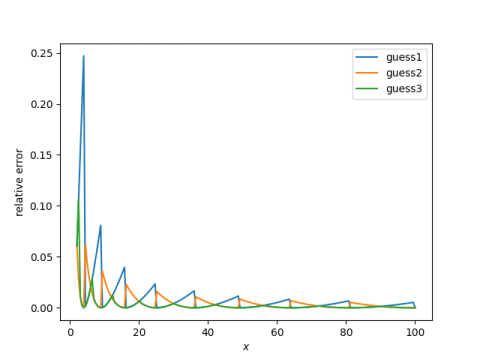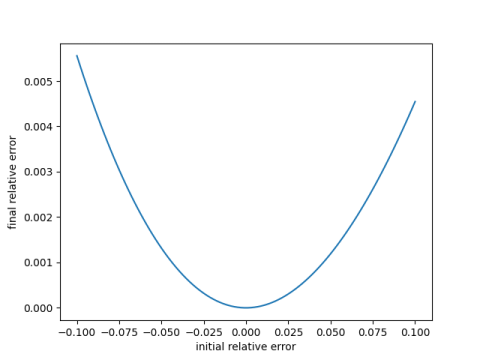# Calculating square roots

Here’s a simple way to estimate the square root of a number x. Take a guess g at the root and compute the average of g and x/g.

If you want to compute square roots mentally or with pencil and paper, how accurate can you get with this method? Could you, for example, get within 1%?

## Initial guess

Obviously the answer depends on your guess. One way to form an initial guess is to round x up to the nearest square and take the root of that as your guess. In symbols, we take ⌈√x⌉ as our guess.

For example, if x = 38, you could take 7 as your guess since 49 is the next square after 38. In that case you’d get

(7 + 38/7)/2 = 6.214

The correct value to three places is 6.164, so our error is about 0.8%.

But we could do better. 38 is much closer to 36 than to 49, so we could take 6 as our initial guess. That is, ⌊√38⌋. Then we have

(6 + 38/6) = 6.167

and our relative error is less than 0.04%.

We’ll look at three strategies:

1. ⌊√x
2. ⌈√x
3. ⌊√x⌋ or ⌈√x

In strategy 3, we choose the guess whose square is closer to x.

## Initial accuracy

Here’s what we get when we use the method above to estimate the square roots of the numbers from 1 to 100.Guess 1 has maximum error 15.5%, where as guess 2 and guess 3 have maximum error 6%.

Guess 2, taking the ceiling, is generally better than guess 1, taking the floor. But guess 3, taking the better of the two, is even better.

More important than which of the three methods used to find the initial guess is being closer to the far end of the range. We could assume x is between 25 and 100 if we first multiply x by a square if necessary to get it into that range. To find the square root of 13, for example, we multiply by 4 and calculate the square root of 13 as half the square root of 52.

If we assume x is between 25 and 100, the maximum errors for the three initial guess methods are 1.4%, 1.3%, and 0.4%.

So to answer our initial question, yes, you can get relative error less than 1%, but you have to do a couple things. You have to multiply by a square to get your number into the range [25, 100] and you have to use the third method of guessing a starting approximation.

## Initial and final error

You don’t have to move your number into the range [25, 100] if you put a little more effort into your initial guess. In the example of x = 13 mentioned above, multiplying by 4 before taking the square root is essentially the same as taking 7/2 as your initial guess for √13 instead of using 3 or 4 as an initial guess.

In short, our discussion of the range on x was really a discussion of initial error in disguise. The size of x determines the quality of our initial guesses, but the size of x itself doesn’t matter to the relative error.

Here’s a plot of the final error as a function of the error in the initial guess.Notice two things. First, the final error is roughly proportional to the square of the error in the initial guess. Second, it’s a little better to guess high than to guess low, which explains why the second method above for guessing starting points is a little better than the first.

## Iteration

Another way to get more accuracy is to repeat our process. That is, after we find our estimate, we use it as a new guess and apply our method again. For example, suppose we want to compute the square root of 30. We would find

(5 + 30/5)/2 = 5.5
(5.5 + 30/5.5)/2 = 5.47727

which is correct to four decimal places

We can find square roots to any accuracy we wish by applying this method enough times. In fact, what we’re doing is applying a special case Newton’s root-finding method.

We showed above that the error after each iteration is on the order of the square of the previous error. So roughly speaking, each iteration doubles the number of correct decimal places.

## Higher-order roots

See the next post for an analogous method for computing cube roots and nth roots in general.